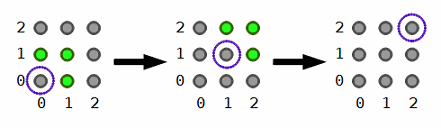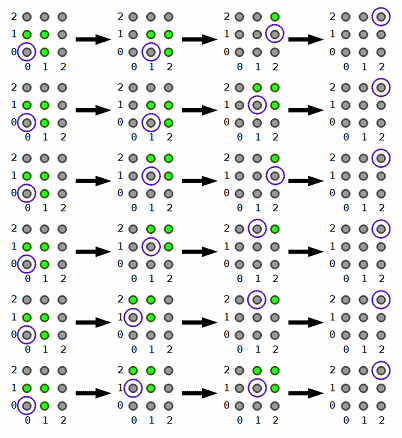### Problem Statement

Fox Ciel likes to jump around in large fields. She is currently at point (0, 0) of a field, and she wants to land at point (Tx, Ty) using exactly R jumps.

In one jump, she can move 0, 1, 2, ..., Mx units in the positive x direction and 0, 1, 2, ..., My units in the positive y direction. All jumps must have a non-zero distance. In addition, there are some jumps which she is not good at. These jumps are described in the int[] bad. If bad contains the number b, it means she cannot make a diagonal jump where she moves exactly b units in the positive x direction and b units in the positive y direction. Each element of bad will be a multiple of 10.

For instance, if Mx=12, My=11, bad={10} and she is at point (0, 0), then the only points she can jump to are the green ones shown below:Return the number of ways she can start at (0, 0), jump exactly R times, and land at (Tx, Ty), modulo 10,007. Two ways are considered to be different if there is an index i, 0 <= i < R, such that Ciel lands at different points after the i-th (0-based) jump in these ways.

### Definition

 Class: FoxJumping Method: getCount Parameters: int, int, int, int, int, int[] Returns: int Method signature: int getCount(int Tx, int Ty, int Mx, int My, int R, int[] bad) (be sure your method is public)

### Constraints

-Tx and Ty will each be between 1 and 800, inclusive.
-Mx and My will each be between 1 and 800, inclusive.
-R will be between 1 and 1,600, inclusive.
-bad will contain between 0 and 50 elements, inclusive.
-Each element of bad will be between 1 and min(Mx, My), inclusive, and be a multiple of 10.
-All elements of bad will be distinct.

### Examples

0)

 `2` `2` `1` `1` `2` `{}`
`Returns: 1`
 There is only 1 way to reach the destination.1)

 `2` `2` `1` `1` `3` `{}`
`Returns: 6`
 The following are the 6 ways she can reach her destination. Note that when she jumps, she must move a distance of at least one unit.2)

 `10` `10` `10` `10` `1` `{}`
`Returns: 1`
 She can jump only once, so there is 1 way to reach the destination.
3)

 `10` `10` `10` `10` `1` `{10}`
`Returns: 0`
 This case is almost the same as the previous one. However, in this case, the required jump is a bad jump, so she cannot reach the destination in a single jump.
4)

 `11` `11` `11` `11` `2` `{10}`
`Returns: 140`
5)

 `123` `456` `70` `80` `90` `{30, 40, 20, 10, 50}`
`Returns: 6723`

#### Problem url:

http://www.topcoder.com/stat?c=problem_statement&pm=11223

#### Problem stats url:

http://www.topcoder.com/tc?module=ProblemDetail&rd=14427&pm=11223

ir5

#### Testers:

PabloGilberto , ivan_metelsky , pieguy

#### Problem categories:

Dynamic Programming, Math